﻿ Power Generation of IMP GeneratorExamples | Product | Murata Software Co., Ltd.# Example5Power Generation of IMP Generator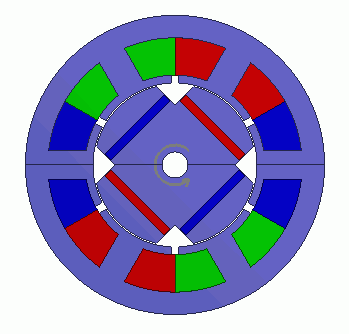General

• Power generation of IPM generator is analyzed.
The power is generated in the coils of the stator by the rotor’s rotation.
The model is a permanent magnet synchronous motor with magnets built in the rotor.

• The current generated by the rotor’s rotation is calculated.

• The analysis is coupled with the external circuit.

• The current and the magnetic flux density are solved.

• Unless specified in the list below, the default conditions will be applied.

### Analysis Conditions

 Item Settings Solver Magnetic Field Analysis [Luvens] Analysis Space 2D Analysis Type Transient Analysis Unit mm Options Select External Circuit Coupling. Select Rotating machinery.   [Conversion] Model Thickness: 30×10^-3[m] Select Partial Model. Number of Divisions of the Whole Model: 2 Circuit Configuration Number of Series: 1 Circuit Configuration Number of parallels: 2 Select Convert the result to the whole model and output it.

The Rotating Machinery tab is set as follows.

 Tab Setting Item Settings Rotating Machinery Rotational Movement Select Constant Velocity Number of Rotations: 3000[r/min] Rotor’s Initial Rotation Position: 0[deg] Number of Slide Mesh Divisions Circumferential Division Angle: 1.0[deg] Rotation per Step: 3[mesh] Number of Slide Mesh Layers: 3

External circuit is as follows.

[R1] is a resistor to detect the generated power. It is placed where a battery is supposed to be placed.

Its value is set to be minute (0.001[Ohm]) for battery’s internal resistance.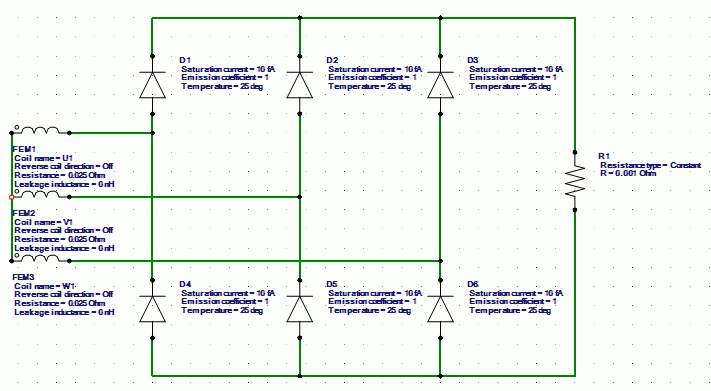Set the Mesh Tab as follows.

 Tab Setting Item Settings Mesh Meshing Setup Automatically set the general mesh size: Deselect General mesh size: 1[mm] Ambient Air Creation Select Create ambient air automatically Ambient Air Scale: 1.25

The Transient Analysis tab is set up as follows.

The umber of steps is 180, circumferential division angle is 1.0[deg], and rotation per step is 3[mesh]. The rotation up to 540 degrees (=180*1.0*3) is analyzed.

Tab

Setting Item

Settings

Transient Analysis

Time step

Automatic

Table

 Number Calculation steps Output steps 1 180 1

### Model

A rotor core and magnet are placed in the center. A stator and coils are placed around them.

This is a 2D model analysis. By utilizing the symmetry of the analysis model, it is made to be a half period symmetric model.

Half period rotation boundary (symmetry) is set.

“Ambient Air Creation” is selected.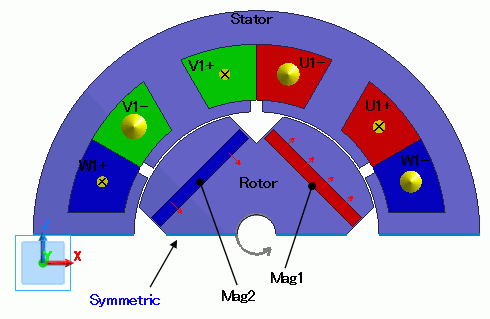### Body Attributes and Materials

 Body Number/Type Body Attribute Name Material Name 6/Sheet Mag1 mag 7/Sheet Mag2 mag 5/Sheet Rotor core 20/Sheet Stator core 22/Sheet U1+ Cu 21/Sheet W1- Cu 25/Sheet V1+ Cu 23/Sheet U1- Cu 26/Sheet W1+ Cu 24/Sheet V1- Cu

The body attribute is set up as follows.

 Body Attribute Name Tab Setting Mag1 Direction Vector: X=1, Y=0, Z=1 Stator/Rotor/Air Rotor Mag2 Direction Vector: X=1, Y=0, Z=-1 Stator/Rotor/Air Rotor Rotor Stator/Rotor/Air Rotor Stator Stator/Rotor/Air Stator U1- Current Waveform: External Circuit Coupling Coil name on the circuit: U1 Turns: 15[Turns] Direction: – Y Direction Stator/Rotor/Air Stator U1+ Current Waveform: External Circuit Coupling Coil name on the circuit: U1 Turns: 15[Turns] Direction: +Y Direction Stator/Rotor/Air Stator V1- Current Waveform: External Circuit Coupling Coil name on the circuit: V1 Turns: 15[Turns] Direction: – Y Direction Stator/Rotor/Air Stator V1+ Current Waveform: External Circuit Coupling Coil name on the circuit: V1 Turns: 15[Turns] Direction: +Y Direction Stator/Rotor/Air Stator W1- Current Waveform: External Circuit Coupling Coil name on the circuit: W1 Turns: 15[Turns] Direction: – Y Direction Stator/Rotor/Air Stator W1+ Current Waveform: External Circuit Coupling Coil name on the circuit: W1 Turns: 15[Turns] Direction: +Y Direction Stator/Rotor/Air Stator

The material properties are set as follows.

Material Name

Tab

Properties

Cu

Conductivity

Conductivity Type: Conductor

Conductivity: 5.977×10^7[S/m]

mag

Permeability

Material Type: Permanent Magnet

Magnet

Magnetization Characteristic Type: Linear

Magnetization Strength: 1.25

Relative Permeability: 1.05

core

Permeability

Magnetization Characteristic Type: Select B-H curve

B-H Curve Table

 Magnetic Field [A/m] Magnetic Flux Density [T] 0 0 58 0.42 90 0.8 180 1.19 380 1.37 1100 1.48 2000 1.55 3000 1.608 11000 1.81 20000 1.91 80000 2.15

### Boundary Conditions

One period symmetric boundary is set.

 Boundary Condition Name/Topology Tab Boundary Condition Type Settings Symmetric Symmetry/Continuity Periodic Rotation Period (1 period)

### Results

The distribution of the magnetic flux density at the rotation angle of 0[deg] is shown below.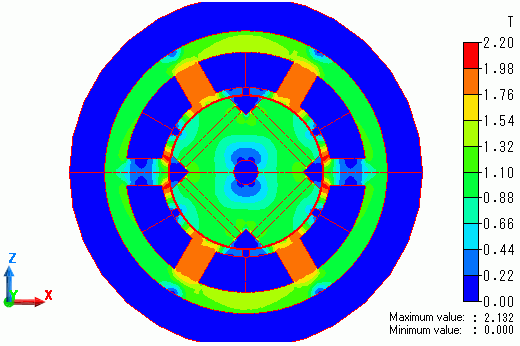In the result table, the generated current is output as the current of circuit component of resistor, which is for detecting the generated power.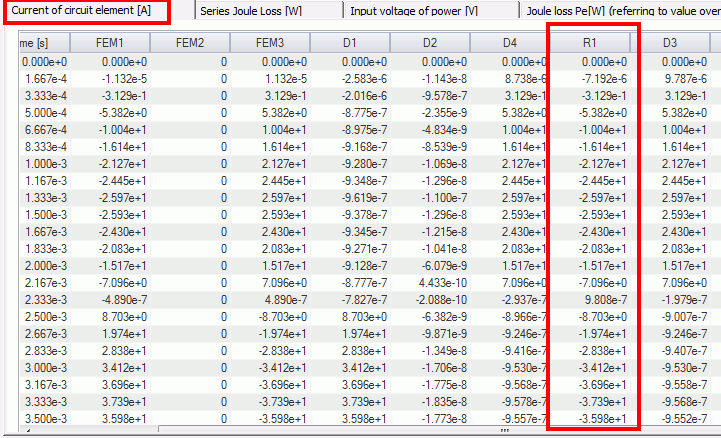The diagram below is time-generated current characteristic.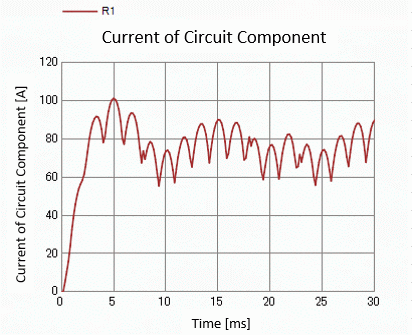The generated power is about 75[A] on average from the current in the steady state.

﻿